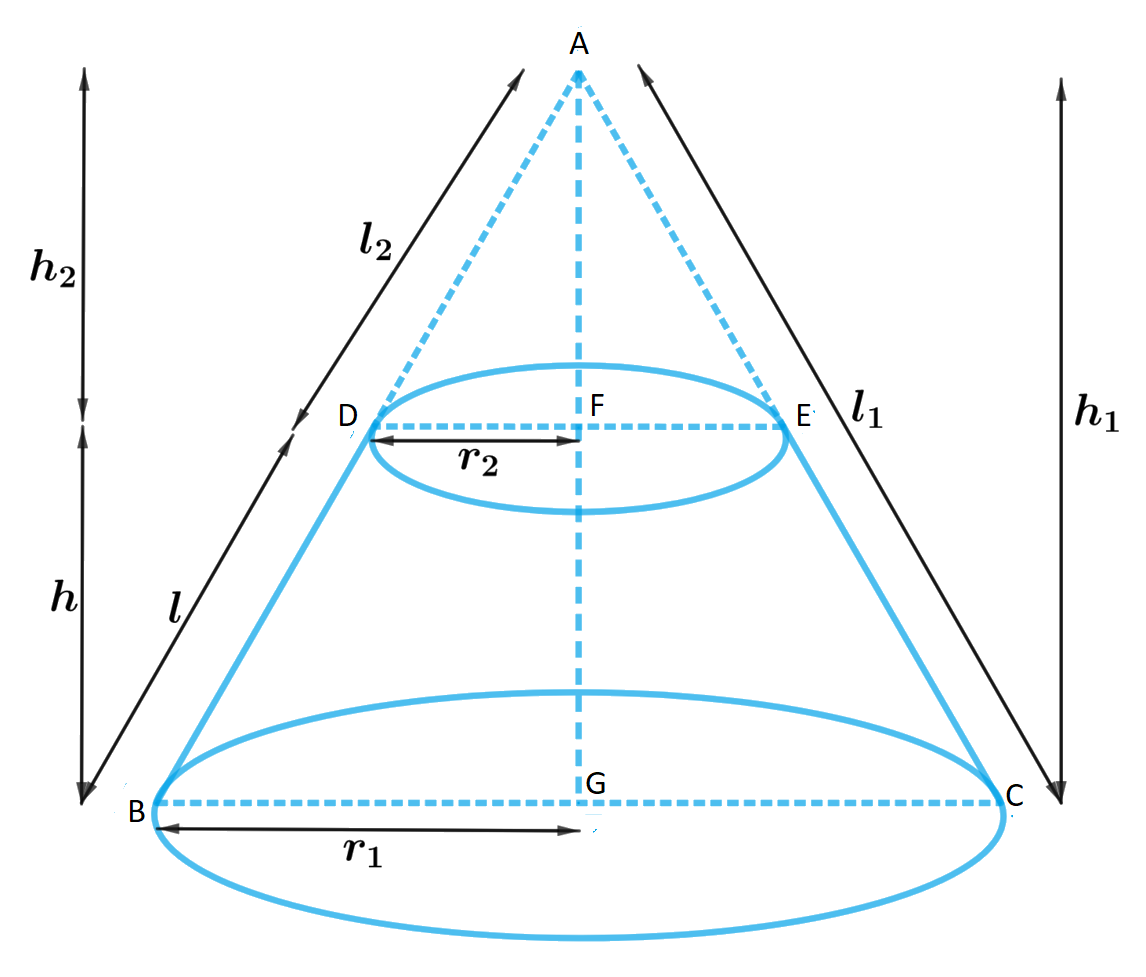# Derive the formula for the curved surface area and total surface area of the frustum of a cone, given to you in Section 13.5, using the symbols as explained.

To do:

We have to derive the formula for the curved surface area and total surface area of the frustum of the cone.

Solution:Let $ABC$ be a cone.

From the cone the frustum $DECB$ is cut by a plane parallel to its base.

$r_1$ and $r_2$ be the radii of the frustum ends of the cone and $h$ be the height of the frustum.

In $\triangle ABG$ and $\triangle ADF$

$DF \| BG$

Therefore,

$\triangle ABG \sim \triangle ADF$

This implies,

$\frac{D F}{B G}=\frac{A F}{A G}=\frac{A D}{A B}$

$\frac{r_{2}}{r_{1}}=\frac{h_{1}-h}{h_{1}}=\frac{l_{1}-l}{l_{1}}$

$\frac{r_{2}}{r_{1}}=1-\frac{h}{h_{1}}=1-\frac{l}{l_{1}}$

$1-\frac{l}{\mathrm{l}_{1}}=\frac{r_{2}}{r_{1}}$

$\frac{l}{l_{1}}=1-\frac{r_{2}}{r_{1}}$

$\frac{l}{l_{1}}=\frac{r_{1}-r_{2}}{r_{1}}$

$\Rightarrow l_{1}=\frac{r_{1} l}{r_{1}-r_{2}}$..............(i)

Curved surface area of frustum $D E C B=$ Curved surface area of cone $A B C$-Curved surface area cone $ADE$

$=\pi r_{1} l_{1}-\pi r_2(l_{1}-l)$

$=\pi r_{1}(\frac{r_{1}l}{r_{1}-r_{2}})-\pi r_{2}[\frac{r_{1}l}{r_{1}-r_{2}}-l]$

$=\frac{\pi r_{1}^{2} l}{r_{1}-r_{2}}-\pi r_{2}(\frac{r_{1}l-r_{1} l+r_{2}l}{r_{1}-r_{2}})$

$=\frac{\pi r_{1}^{2} l}{r_{1}-r_{2}}-\frac{\pi r_{2}^{2}l}{r_{1}-r_{2}}$

$=\pi l[\frac{r_{1}^{2}-r_{2}^{2}}{r_{1}-r_{2}}]$

$=\pi l[\frac{(r_1-r_2)(r_1+r_2)}{r_1-r_2}$

$=\pi (r_1+r_2)l$

The total surface area of frustum $=$ Curved surface area of frustum $D E C B+$ area of upper circle $+$ area of lower circle

$=\pi (r_1+r_2)l+r_1^2+r_2^2$